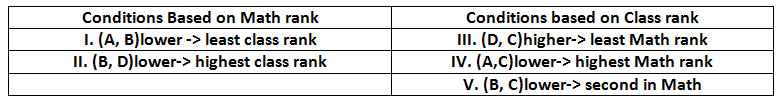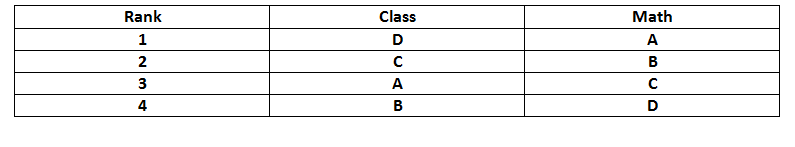# Logical Reasoning Puzzles for CAT Set-2 PDF

0
7415

## Logical Reasoning Puzzles for CAT Set-2 PDF:

Download important logical reasoning (LR) Solved Puzzles Set-2 PDF based on previous asked questions in CAT exam.

Instructions: Four friends A, B, C and D study in the same class in St. Theresa Public school. The first four ranks in the class were obtained by these four students. They also were the top 4 rankers in Mathematics. The following observations are made based on their overall class ranks and ranks of each subject.

(i) The one with the lower rank in Maths between A and B had the least class rank
(ii) The one with the higher class rank between D and C had the least Math rank
(iii) The one with the lower class rank between B and C, had a better Math rank than exactly two other friends
(iv) The one with the lower Math rank between B and D, had the highest class rank
(v) The one with lower class rank between A and C had the highest Math rank

Note: A lower numerical rank is considered as a higher rank

Question 1:

How many different combinations are possible for the class rank?

a) 1
b) 2
c) 3
d) 4

Question 2:

Who got the third rank in the class?

a) A
b) B
c) C
d) D

Question 3:

The exact Math ranks of how many people can be uniquely determined?

a) 1
b) 2
c) 3
d) 4

Question 4:

Who obtained the third rank in Maths ?

a) A
b) B
c) C
d) cannot be determined

Question 5: How many friends obtained the same rank for the math and class rank?

a) 0
b) 1
c) 2
d) cannot be determined

Answers & Solutions for Logical Reasoning Puzzles for CAT Set-2 PDF:

Answers & Solutions (1 – 5):

First let’s write down the conditions given for Class rank and Math rank separately.We see A and B are present in both the columns. Thus, making assumptions based on them will yield the most result. Let’s first assume A has a lower Math rank than B.

From I, A has the least class rank. Thus, from IV, A has the highest Math rank. This contradicts our assumption. Thus, A definitely has a higher Math rank than B.

Thus, from I. B is 4th in Class rank. From V. B is the second in Math. Since A has higher Math rank than B, A will be the 1st in Math. Applying II, we get D is the 1st in the Class. Further using conditions III and IV, the following table can be formed.1) Answer (A)